Ex 12.3

Chapter 12 Class 10 Areas related to Circles
Serial order wise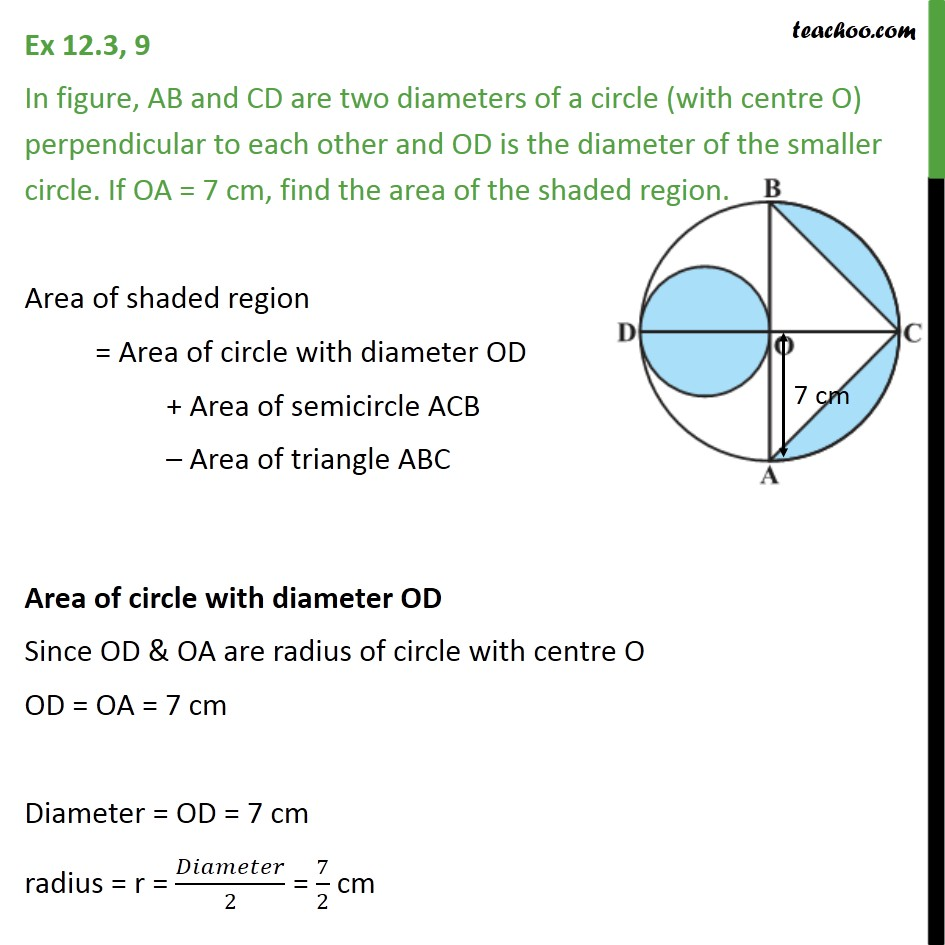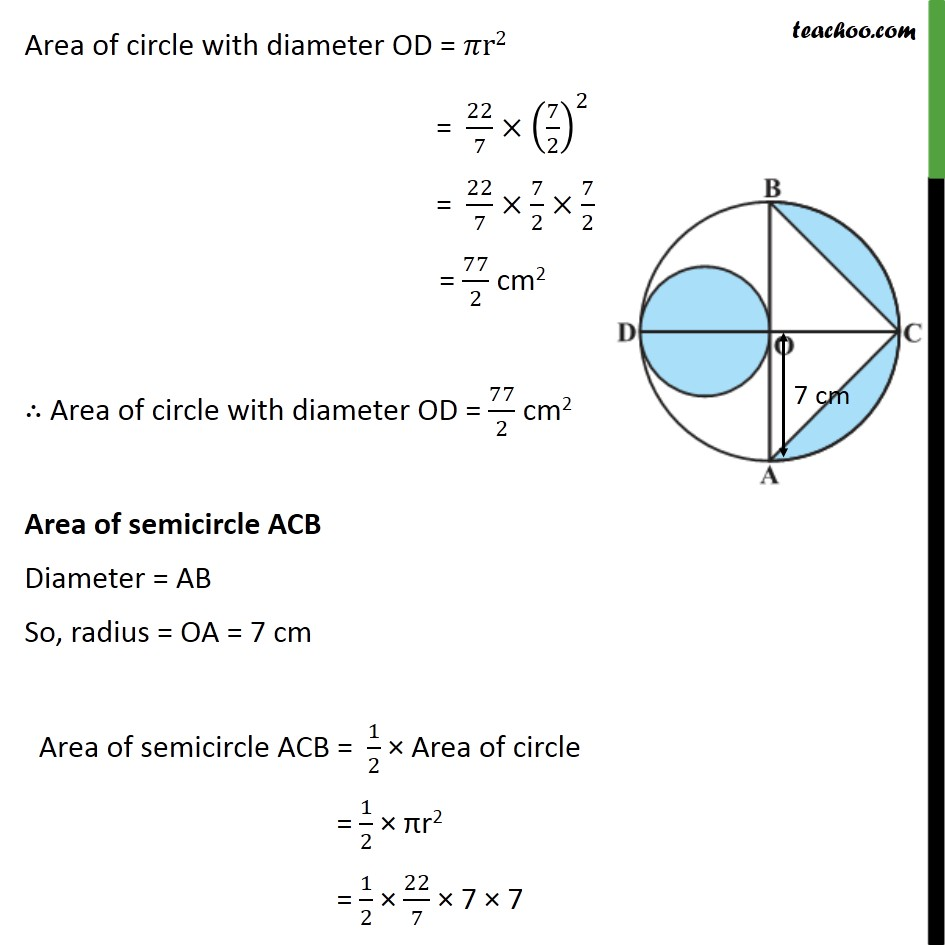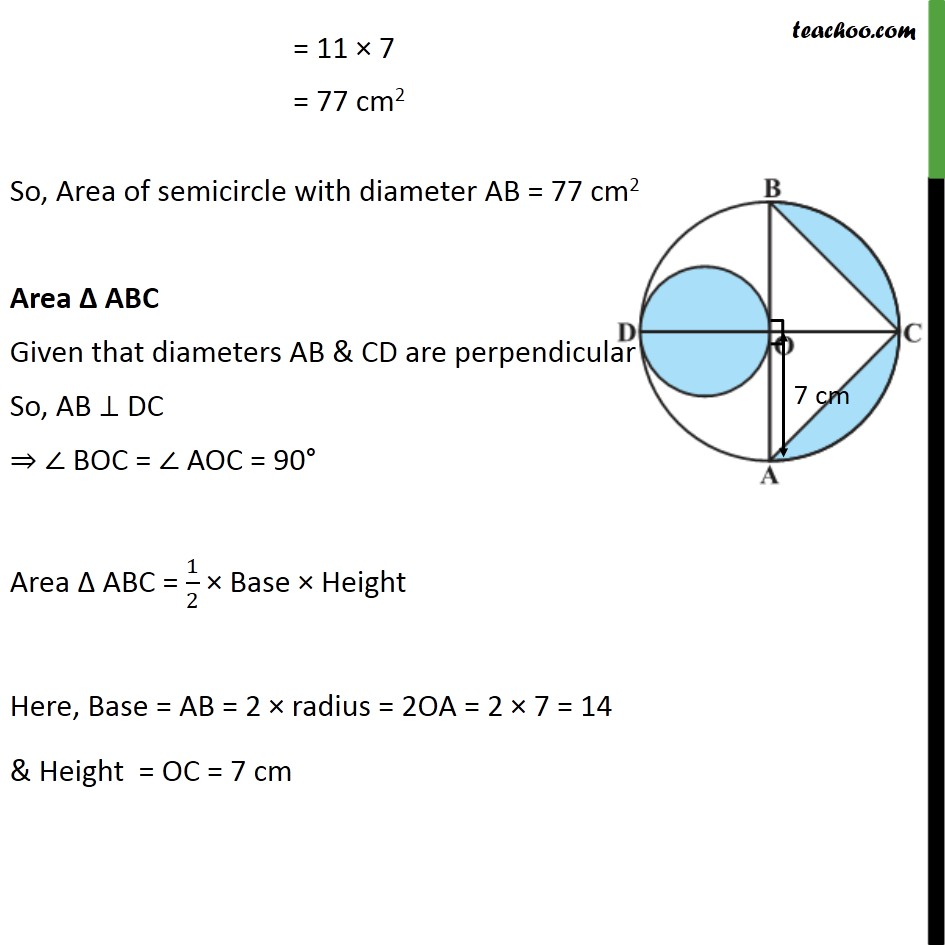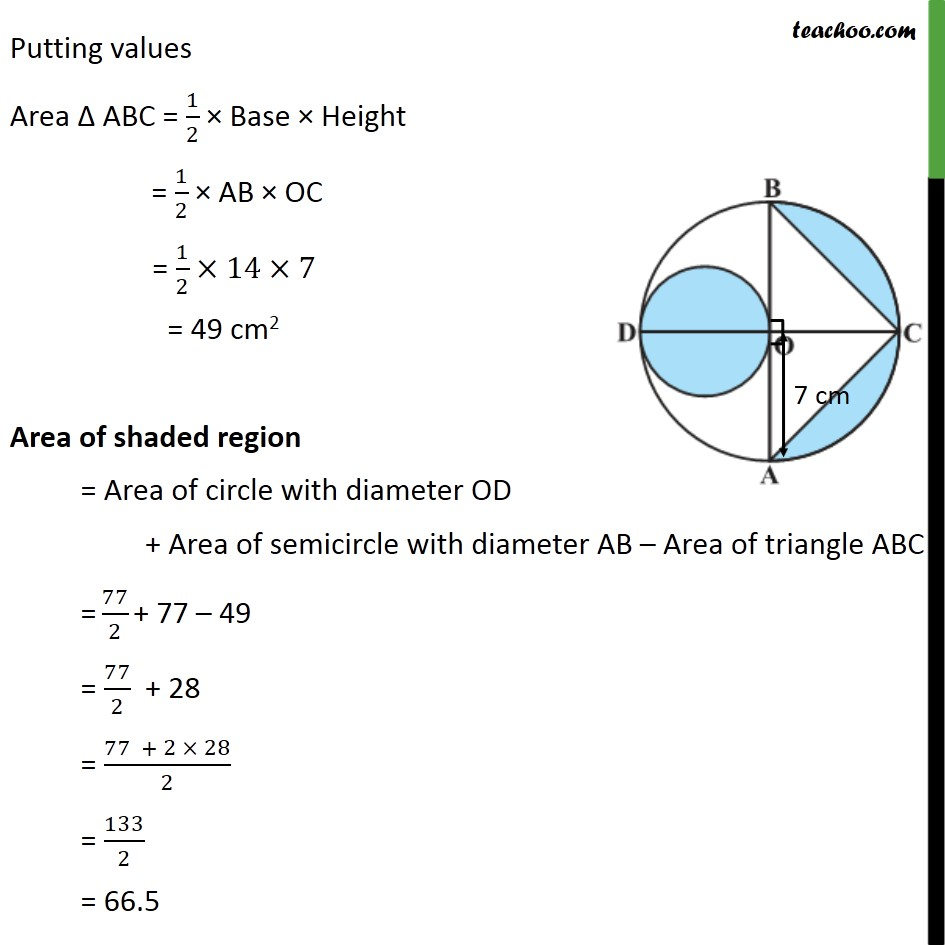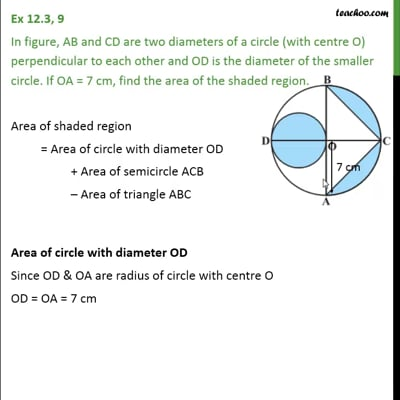This video is only available for Teachoo black users

Solve all your doubts with Teachoo Black (new monthly pack available now!)

### Transcript

Ex 12.3, 9 In figure, AB and CD are two diameters of a circle (with centre O) perpendicular to each other and OD is the diameter of the smaller circle. If OA = 7 cm, find the area of the shaded region. Area of shaded region = Area of circle with diameter OD + Area of semicircle ACB Area of triangle ABC Area of circle with diameter OD Since OD & OA are radius of circle with centre O OD = OA = 7 cm Diameter = OD = 7 cm radius = r = /2 = 7/2 cm Area of circle with diameter OD = r2 = 22/7 (7/2)^2 = 22/7 7/2 7/2 = 77/2 cm2 Area of circle with diameter OD = 77/2 cm2 Area of semicircle ACB Diameter = AB So, radius = OA = 7 cm Area of semicircle ACB = 1/2 Area of circle = 1/2 r2 = 1/2 22/7 7 7 = 11 7 = 77 cm2 So, Area of semicircle with diameter AB = 77 cm2 Area ABC Given that diameters AB & CD are perpendicular So, AB DC BOC = AOC = 90 Area ABC = 1/2 Base Height Here, Base = AB = 2 radius = 2OA = 2 7 = 14 & Height = OC = 7 cm Putting values Area ABC = 1/2 Base Height = 1/2 AB OC = 1/2 14 7 = 49 cm2 Area of shaded region = Area of circle with diameter OD + Area of semicircle with diameter AB Area of triangle ABC = 77/2 + 77 49 = 77/2 + 28 = (77 + 2 28)/2 = 133/2 = 66.5